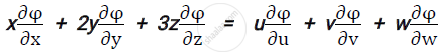BE Chemical Engineering Semester 1 (FE First Year)University of Mumbai
Share
Notifications

View all notifications
Books Shortlist
Your shortlist is empty

# Question Paper Solutions for Applied Mathematics 1 CBCGS 2016-2017 BE Chemical Engineering Semester 1 (FE First Year)

Login
Create free account

Forgot password?
SubjectApplied Mathematics 1
Year2016 - 2017 (December)
Applied Mathematics 1
CBCGS
2016-2017 December
Marks: 80

(1) Question no. 1 is compulsory.
(2) Attempt any 3 questions from remaining five questions.

1
1.a

If cos alpha cos beta=x/2, sinalpha sinbeta=y/2, prove that:

sec(alpha -ibeta)+sec(alpha-ibeta)=(4x)/(x^2-y^2)

Chapter:  Complex Numbers
Concept: Review of Complex Numbers‐Algebra of Complex Number
1.b

If z =log(e^x+e^y) "show that rt" - s^2 = 0  "where r"= (del^2z)/(delx^2),t=(del^2z)/(dely^2)"s"=(del^2z)/(delx dely)

Chapter:  Complex Numbers
Concept: Review of Complex Numbers‐Algebra of Complex Number
1.c

If x = uv, y =(u+v)/(u-v).find (del(u,v))/(del(x,y)).

Chapter:  Complex Numbers
Concept: Review of Complex Numbers‐Algebra of Complex Number
1.d

If y=2^xsin^2x cosx find y_n

Chapter:  Complex Numbers
Concept: Review of Complex Numbers‐Algebra of Complex Number
1.e

Express the matrix as the sum of symmetric and skew symmetric matrices.

Chapter:  Matrices
Concept: Addition of a Matrix
1.f

Evaluat lim_(x->0) (e^(2x)-(1+x)^2)/(xlog(1+x)

Chapter:  Indeterminate Forms, Numerical Solutions of Transcendental Equations and System of Linear Equations
Concept: L‐ Hospital Rule
2
2.a

Show that the roots of x5 =1 can be written as 1, alpha^1,alpha^2,alpha^3,alpha^4 .hence show that (1-alpha^1) (1-alpha^2) (1-alpha^3)(1-alpha^4)=5.

Chapter:  Complex Numbers
Concept: Powers and Roots of Trigonometric Functions
2.b

Reduce the following matrix to its normal form and hence find its rank.

Chapter:  Matrices
Concept: Reduction to Normal Form
2.c

Solve the following equation by Gauss-Seidel method upto four iterations

4x-2y-z=40, x-6y+2y=-28, x-2y+12z=-86.

Chapter:  Indeterminate Forms, Numerical Solutions of Transcendental Equations and System of Linear Equations
Concept: Gauss Seidal Iteration Method
3
3.a

Investigate for what values of μ and λ the equations x+y+z=6, x+2y+3z=10, x+2y+λz=μ has
1) No solution
2) A unique solution
3) Infinite number of solutions.

Chapter:  Matrices
Concept: consistency and solutions of homogeneous and non – homogeneous equations
3.b

If u=x^2+y^2+z^2 where x=e^t, y=e^tsint,z=e^tcost

Prove that (du)/(dt)=4e^(2t)

Chapter:  Complex Numbers
Concept: Review of Complex Numbers‐Algebra of Complex Number
3.c

Show that sin(e^x-1)=x^1+x^2/2-(5x^4)/24+...................

Chapter:  Applications of Partial Differentiation , Expansion of Functions
Concept: Expansion of 𝑒^𝑥 , sin(x), cos(x), tan(x), sinh(x), cosh(x), tanh(x), log(1+x), 𝑠𝑖𝑛−1 (𝑥),𝑐𝑜𝑠−1 (𝑥),𝑡𝑎𝑛−1 (𝑥)
3.d

Expand 2x^3+7x^2+x-6 in powers of (x-2)

Chapter:  Complex Numbers
Concept: Expansion of sinnθ, cosnθ in powers of sinθ, cosθ
4
4.a

If x = u+v+w, y = uv+vw+uw, z = uvw and φ is a function of x, y and z
Prove thatChapter:  Partial Differentiation
Concept: Euler’s Theorem on Homogeneous functions with two and three independent variables (with proof)
4.b

If tan(θ+iφ)=tanα+isecα
Prove that
1)e^(2varphi)=cot(varphi/2)
2) 2theta=npi+pi/2+alpha

Chapter:  Partial Differentiation
Concept: Euler’s Theorem on Homogeneous functions with two and three independent variables (with proof)
4.c

Find the roots of the equation x^4+x^3 -7x^2-x+5 = 0 which lies between 2 and 2.1 correct to 3 places of decimals using Regula Falsi method.

Chapter:  Indeterminate Forms, Numerical Solutions of Transcendental Equations and System of Linear Equations
Concept: Regula – Falsi Equation
5
5.a

If y=(x+√x2-1 ,Prove that

(x^2-1)y_(n+2)+(2n+1)xy_(n+1)+(n^2-m^2)y_n=0

Chapter: [6.01] Successive Differentiation
Concept: Leibnitz’S Theorem (Without Proof) and Problems
5.b

Using the encoding matrix [(1,1),(0,1)] encode and decode the messag I*LOVE*MUMBAI.

Chapter:  Matrices
Concept: Application of Inverse of a Matrix to Coding Theory
5.c

Considering only principal values separate into real and imaginary parts

i^((log)(i+1))

Chapter: [6.02] Logarithm of Complex Numbers
Concept: Separation of Real and Imaginary Parts of Logarithmic Functions
5.d

Show that ilog((x-i)/(x+i))=pi-2tan6-1x

Chapter: [6.02] Logarithm of Complex Numbers
Concept: Logarithmic Functions
6
6.a

Using De Moivre’s theorem prove that]

cos^6theta-sin^6theta=1/16(cos6theta+15cos2theta)

Chapter:  Complex Numbers
Concept: D’Moivre’S Theorem
6.b

If u =sin^(-1)((x^(1/3)+y^(1/3))/(x^(1/2)-y^(1/2))), Prove that

x^2(del^2u)/(delx^2)+2xy(del^2u)/(delxdely)+y^2(del^2u)/(dely^2)=tanu/144(tan^2u+13)

Chapter:  Matrices
Concept: System of Homogeneous and Non – Homogeneous Equations
6.c

Find the maxima and minima of x^3 y^2(1-x-y)

Chapter:  Applications of Partial Differentiation , Expansion of Functions
Concept: Maxima and Minima of a Function of Two Independent Variables

#### Request Question Paper

If you dont find a question paper, kindly write to us

View All Requests

#### Submit Question Paper

Help us maintain new question papers on shaalaa.com, so we can continue to help students

only jpg, png and pdf files

## University of Mumbai previous year question papers Semester 1 (FE First Year) Applied Mathematics 1 with solutions 2016 - 2017

University of Mumbai Semester 1 (FE First Year) Applied Mathematics 1 question paper solution is key to score more marks in final exams. Students who have used our past year paper solution have significantly improved in speed and boosted their confidence to solve any question in the examination. Our University of Mumbai Semester 1 (FE First Year) Applied Mathematics 1 question paper 2017 serve as a catalyst to prepare for your Applied Mathematics 1 board examination.
Previous year Question paper for University of Mumbai Semester 1 (FE First Year) Applied Mathematics 1-2017 is solved by experts. Solved question papers gives you the chance to check yourself after your mock test.
By referring the question paper Solutions for Applied Mathematics 1, you can scale your preparation level and work on your weak areas. It will also help the candidates in developing the time-management skills. Practice makes perfect, and there is no better way to practice than to attempt previous year question paper solutions of University of Mumbai Semester 1 (FE First Year).

How University of Mumbai Semester 1 (FE First Year) Question Paper solutions Help Students ?
• Question paper solutions for Applied Mathematics 1 will helps students to prepare for exam.
• Question paper with answer will boost students confidence in exam time and also give you an idea About the important questions and topics to be prepared for the board exam.
• For finding solution of question papers no need to refer so multiple sources like textbook or guides.
S